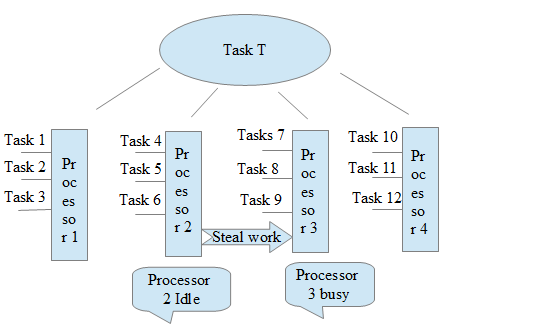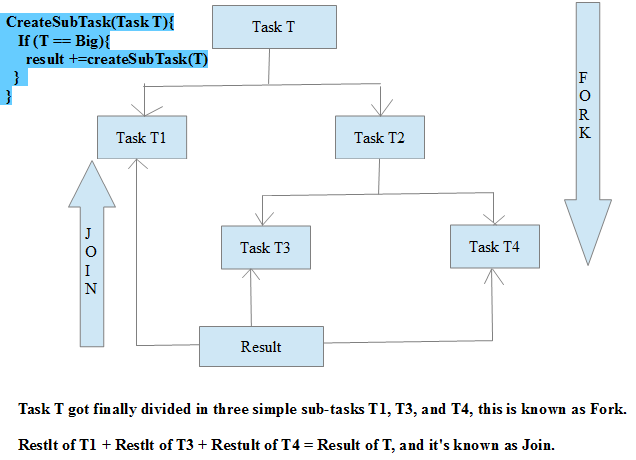{{announcement.body}}
{{announcement.title}}

# A Look at ForkJoinPool and Parallel Streams

DZone 's Guide to

# A Look at ForkJoinPool and Parallel Streams

### The ForkJoin pool was put into place to support parallel streams. Get a look at how the pool works and how the work-stealing algorithm help divide up tasks.

· Java Zone ·
Free Resource

Comment (4)

Save
{{ articles.views | formatCount}} Views

The Common ForkJoin Pool was introduced in Java 8 to support parallel streams, CompletableFuture, etc. ForkJoin is based on parallel computing, where a problem gets divided into sub-problems until the sub-problems are simple enough to solve simultaneously in separate threads, after which the results are aggregated.

The algorithm that's been used in ForkJoin implementations is the 'Work-Stealing Algorithm' to ensure that no CPU is idle.

Let's take a look at work-stealing algorithm.

Suppose there are four processors on a system and Task T has been divided into 12 subtasks, shown below, from T1 to T12.

Each processor has three subtasks. But suppose Processor 3 is too busy with work and Processor 2 is idle—it either finished all the tasks or it's in a waiting state. Then, Processor 2 will ask to Processor 3 if it needs help and takes a percentage of the tasks. So, Processor 2 is 'stealing the work' from Processor 3.

The below snapshot depicts this process:Now let's look at the snapshot below to understand the ForkJoin framework:So above snapshot shows the recursive approach to divide the task until subtask is a simple task.

We create an instance of ForkJoinPool as below -

`ForkJoinPool pool = new ForkJoinPool(parallelism);`

Here, parallelism is the target parallelism level (number of processors), which can be seen below:

`Runtime.getRuntime().availableProcessors(); // returns 4 on my system`

To support parallelism for collections using parallel streams, a common ForkJoinPool is used internally.

We can get a common pool using the ForkJoin static method below:

`ForkJoinPool commonPool = ForkJoinPool.commonPool();`

To see the parallelism level for this commonPool, use:

`commonPool.getParallelism(); //returns 3 on my system`

The ForkJoin framework provides two types of tasks: 'RecursiveTask' and 'RecursiveAction'.  The difference between these two is that RecursiveTask can return a value while RecursiveAction cannot.

Let's see an example using RecursiveTask.

FibonacciComutation.java:

``````package arun.test.forkjoin;

import org.apache.commons.logging.Log;
import org.apache.commons.logging.LogFactory;

/**
* @author arun.pandey
*/
public class FibonacciComputation extends RecursiveTask<Integer> {
public static final Log LOG = LogFactory.getLog(ForkJoinTest.class);
private final int number;

public FibonacciComputation(int number) {
this.number = number;
}

@Override
public Integer compute() {
if (number <= 1)
return number;
FibonacciComputation f1 = new FibonacciComputation(number - 1);
f1.fork();
FibonacciComputation f2 = new FibonacciComputation(number - 2);
return f2.compute() + (Integer)f1.join();
}
}
``````

The client code is shown below:

FibonacciComutationClient.java:

``````package arun.test.forkjoin;

import java.util.concurrent.ForkJoinPool;
import org.apache.commons.logging.Log;
import org.apache.commons.logging.LogFactory;

/**
* @author arun.pandey
*/
public class FibonacciComutationClient {
public static final Log LOG = LogFactory.getLog(FibonacciComutationClient.class);

public static void main(String args[]){
//to calculate 20th element of Fibonacci-Series
int number = 20;
int poolSize = Runtime.getRuntime().availableProcessors();
ForkJoinPool pool = new ForkJoinPool(poolSize);
long beforeTime = System.currentTimeMillis();
LOG.info("Parallelism  => "+ pool.getParallelism());

Integer result = (Integer) pool.invoke(new FibonacciComputation(number));

LOG.info("Total Time in MilliSecond Taken ->  "+ (System.currentTimeMillis() - beforeTime));
LOG.info(number +"the element of Fibonacci Number = "+result);
}
}
``````

If you execute the client code, you can see the execution time lesser than a normal recursive Fibonacci series implementation. Since it's dividing into subtasks it's a bit faster.

I hope this gives you a better understanding of the ForkJoin framework. Happy learning!

Topics:
concurrency, forkjoin, java, parallel streams, tutorial

Comment (4)

Save
{{ articles.views | formatCount}} Views

Opinions expressed by DZone contributors are their own.